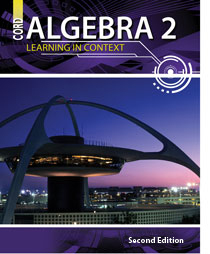# Algebra 2 - 2nd Edition

## Chapter 12: Conic Sections

Some links are repeated for use with more than one lesson.

### These links are for use with the entire chapter.

http://mathforum.org/~sanders/geometry/GP16Conics.html
This series of pages contains a look at all four conics and a link to look at real-world applications. (Click Go to... links at bottom of each page.)

http://quizlet.com/set/8823/

### 12.1 Distance and Midpoint Formulas

http://www.algebralab.org/studyaids/studyaid.aspx?file=Algebra1_13-1.xml
This page contains a lesson on the distance and midpoint formulas and practice problems.

http://www.explorelearning.com/index.cfm?method=cResource.dspDetail&ResourceID=64
This page contains a gizmo activity on the distance formula. This website requires Shockwave Player to run some of its features.

http://www.purplemath.com/modules/midpoint.htm
This series of pages presents and demonstrates the application of the midpoint formula to solve problems.

http://hotmath.com/help/gt/genericalg2/section_12_1.html
This page provides several practice problems using the distance and midpoint formulas.

### 12.2 General Conics

http://cs.jsu.edu/mcis/faculty/leathrum/Mathlets/conics.html
This page contains a conic graphing applet that allows the user to change values of the coefficients. This website requires Java to run some of its features.

http://math2.org/math/algebra/conics.htm

### 12.3 Parabolas

http://www.ies-math.com/math/java/conics/draw_parabola/draw_parabola.html
This page contains an applet that demonstrates the relationship between the focus and directrix. This website requires Java to run some of its features.

http://www.ies-math.com/math/java/conics/focus/focus.html
This page contains an applet that visually demonstrates the relationship between the focus and parameters of the parabola. This website requires Java to run some of its features.

http://www.coolmath.com/algebra/25-conic-sections/03_parabolas-intro.htm
This page contains an explanation of the properties of parabolas with animated diagrams.

http://www.regentsprep.org/regents/math/algebra/ac4/indexAC4.htm
This series of pages demonstrates several techniques for graphing and understanding parabolic functions.

http://www.purplemath.com/modules/parabola.htm
This series of pages teaches about the general formula for parabolas, and how to use it with several examples involving the foci locations, vertex, directrix, and so forth.

### 12.4 Ellipses

http://www.analyzemath.com/EllipseEq/EllipseEq.html

http://www.mathsisfun.com/geometry/ellipse.html
This page discusses and demonstrates many aspects about ellipses, such as the foci, the major and minor axes, area, and general equation, including some practice problems.

http://www.purplemath.com/modules/ellipse.htm
This series of pages teaches about the general formula for ellipses, and how to use it with several examples involving the foci locations, eccentricity, and so forth.

http://www.coolmath.com/algebra/25-conic-sections/02_ellipses-intro.htm

### 12.5 Circles

http://www.analyzemath.com/CircleEq/CircleEq.html
This page contains a tutorial on graphing circles and an interactive applet.

http://www.regentsprep.org/Regents/math/algtrig/ATC1/indexATC1.htm

http://www.purplemath.com/modules/circle.htm
This series of pages teaches about the general formula for circles, and how to use it with several examples involving the radius, center location, and so forth.

### 12.6 Hyperbolas

http://www.coolmath.com/algebra/25-conic-sections/04_hyperbolas-intro.htm
This page contains an introduction to hyperbolas, including real world examples and animated diagrams.

http://www.mathwarehouse.com/hyperbola/graph-equation-of-a-hyperbola.php
This page contains explanations and practice problems solving and interpreting the graphs of hyperbolas.

http://www.ies-math.com/math/java/conics/draw_hyper/draw_hyper.html
This page contains an applet that demonstrates the relationship between the focus and graph of the hyperbola. This website requires Java to run some of its features.

http://www.purplemath.com/modules/hyperbola.htm
This series of pages teaches about the general formula for hyperbolas, and how to use it with several examples involving the asymptotes, vertices, foci, eccentricity, and so forth.